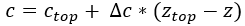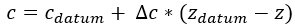# Undrained

For the Undrained soil model, the friction angle phi is automatically set to zero. The shear strength is defined only by the cohesion of the material. Three sub-options for defining the Undrained cohesion are available, by selecting from the Cohesion Type drop-list: Constant, F(Depth), F(Datum).

## Constant

Cohesion is constant throughout the material.

## F(Depth)

Cohesion is a function of depth, where depth is measured from the top of the material layer, to the center of a column base.• Cohesion (Top) is the Cohesion at the top of the material layer.
• Cohesion Change is the rate of change of Cohesion with depth.
• If you wish to specify a maximum soil strength, select the Cutoff checkbox and enter a maximum value for Cohesion. If the rate of Cohesion Change is negative, then the Cutoff value represents the minimum soil strength.

## F(Datum)

Cohesion is a function of depth, where depth is measured from a user-specified Datum (z-coordinate) to the center of a column base.• Datum is the datum elevation (z-coordinate).
• Cohesion (Datum) is the Cohesion at the Datum elevation.
• Cohesion Change is the rate of change of Cohesion with distance (zdatum-z) from the Datum. If the Cohesion Change is positive, then cohesion increases below the datum and decreases above the datum, according to the change in elevation zdatum-z.
• If you wish to specify a maximum soil strength, select the Cutoff checkbox and enter a maximum value for Cohesion. If the rate of Cohesion Change is negative, then the Cutoff value represents the minimum soil strength.

## Tensile Strength

See the Tensile Strength topic.